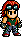# Sergei Dragunov/Tekken 5: Dark Resurrection MovelistLooks like Hwoarang has run off and made this page, Sergei Dragunov/Tekken 5: Dark Resurrection Movelist, without telling Baek Doo San and hasn't done it right!Please fix it by re-formatting this page to follow it's [[Template:{{{1}}}|template]].

Dragunov/Tekken 5: Dark Resurrection Moves List is a list of all of the moves that Sergei Dragunov can perform in Tekken 5: Dark Resurrection.

## §Moves List

1. Eye Gouge to Hilt: Square Square X.
2. Jab to Body Blow: Square Triangle.
3. Salvo: Square Triangle Square.
4. Hammer and Sickle: Square X Triangle.
5. One Two Scud Kick: Triangle Square X.
6. One Two Mine Kick: Triangle Square Circle.
7. Karnov Kick: Circle X.
8. Iron Flail: X + Circle.
9. Stun Gun: East Triangle Circle.
10. High Salute: East X.
11. Cougar Slash: East Circle Circle.
12. Cougar Maul: East Circle South Circle.
13. Scabbard: East Square + Triangle.
15. Scimitar: Southeast Triangle.
16. Mantis Heal: Southeast X.
17. Bunker-buster Elbow: South Square.
18. Razer: South Triangle.
19. March of Tyranny: South X Circle.
20. Separator: South Circle Square X.
21. Heavy Separator: South Circle Circle X.
22. Death's Door: Southwest Triangle Square Triangle.
23. Clipping Sweep: Southwest X.
24. Clipping Sweep to Feint & Catch: (Approach (crouching enemy)) Southwest X Square + Triangle.
25. Eye Blast: West Square.
26. Eye Gouge to Stingray: West Triangle Square X.
27. Back Lock Kick to Ear Grab: West X Square.
28. Death March to Ear Grab: West Circle Triangle Square.
29. Death March to Takedown: West Circle Triangle Square + Triangle.
30. War Machine: West Circle X.
31. Blizzard Hammer: West Square + Triangle.
32. Inertia Kick: West X + Circle.
33. Sub-Zero: West X + Triangle.
34. Counterstrike: Northwest Triangle.
35. Falcon Dive: Northeast Triangle.
36. Slicing Sobat: Northeast Circle.
37. Scorpion Scissors: Northeast X + Circle.
38. Russian Sickle: East East (hold) Triangle.
39. Feint & Catch: East East (hold) Triangle Square + Triangle.
40. Stinger Kick: East East (hold) X.
41. Rolling Thunder: East East (hold) Circle.
42. Stinger Elbow: South Southeast East (hold) Triangle.
43. Snap Knee: South Southeast East (hold) Circle.
44. Pinpoint Fracture: South Southeast East (hold) Circle (during hit) Square X Circle Triangle (or Triangle Circle X Square).
45. Mass Elbow: South Southwest West Triangle.
46. Russian Assault: East East East Triangle.
47. Spetnaz Assault: East East East Circle.
48. Double Hilt: (While rising) Square X.
49. Ballistic Upper: (While rising) Triangle.
50. Glacial Hilt: (While rising) X.
51. Frost Tackle: (While rising) Square + Triangle.
52. Integral Slicer: (During sidestep) Triangle X.
53. Integral Blitz: (During sidestep) Triangle Square + Triangle Circle.
54. Integral Takedown: (During sidestep) Triangle Square + Triangle Square + Triangle.
55. Cane Stab: (Back towards enemy) South X.
56. Living Dead: (While down (facing down)) Square + Triangle.
57. Six Feet Under: (While enemy is down) South X + Circle.
58. 10 Hit Combo 1: Square X Square Triangle Square Square Circle Square X Triangle.
59. 10 Hit Combo 2: Square X Square Triangle Circle Square + Triangle.
60. Volk Clutch: (Approach enemy) Square + X (or East Square + X).
61. Purge Slam: (Approach enemy) Triangle + Circle (or East Triangle + Circle).
62. Tarantula Sting: (Approach from left side) Square + X (or Triangle + Circle).
63. Reverse Fracture: (Approach from right side) Square + X (or Triangle + Circle).
64. Absolute Silence: (Approach from behind) Square + X (or Triangle + Circle).
65. Reverse Victor Clutch: (Approach enemy) East Square + Circle.
66. Victor Clutch: (Approach enemy) East Triangle + X.
67. Blizzard Rush: (Approach enemy) East East (hold) Square + Triangle.
68. Cold Fate: (Approach (crouching enemy)) South Square + X (or South Triangle + Circle).
69. Ambush Tackle: Southwest Square + Triangle (or South Square + Triangle).
70. Frost Bite: (During tackle) Square.
71. Achilles' Hold: (During tackle) Triangle.
72. Iron Curtain: (During tackle) Square + Triangle.
73. Red Alert: (Time with enemy attack) West Square + X (or West Triangle + Circle).